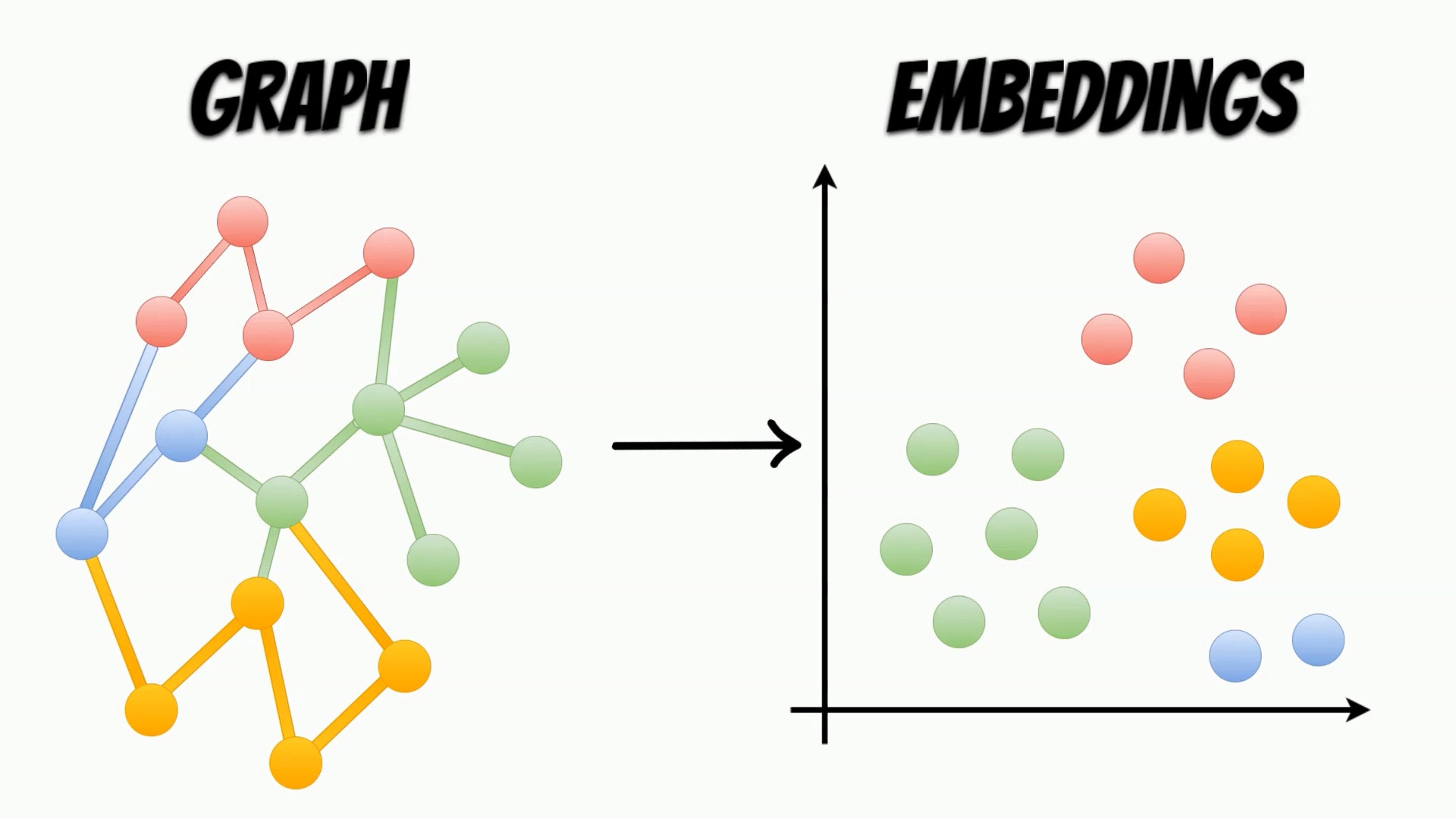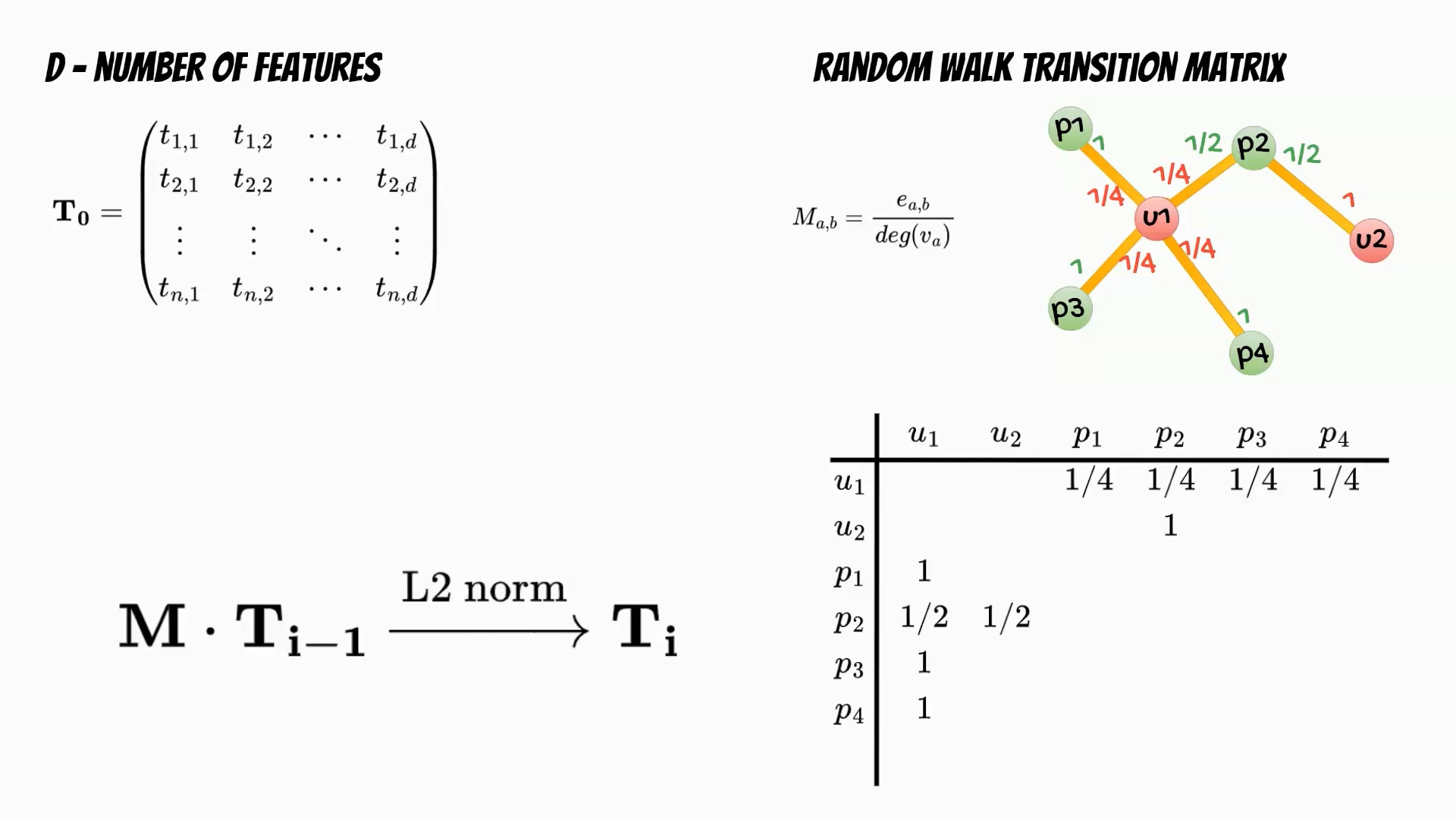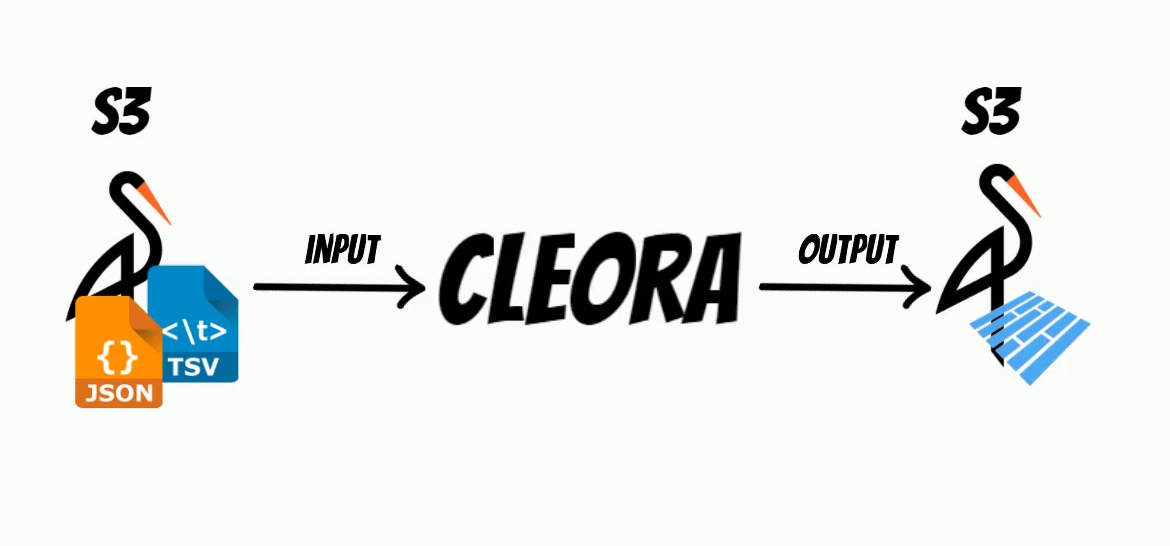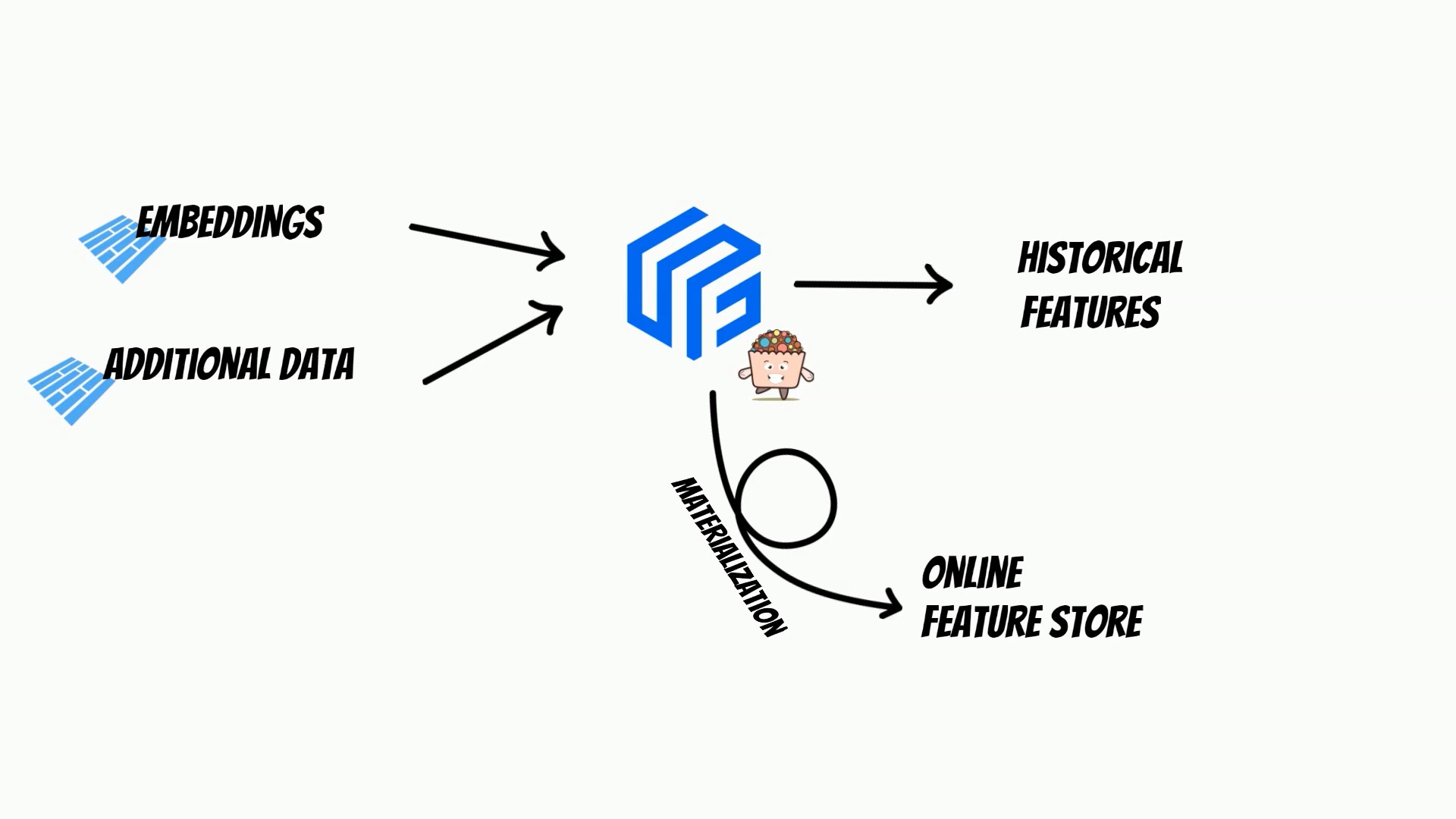# Graph Embeddings with Feature StoreIn this video I will show how to generate and use graph embeddings with feature store.

Graphs are structures, which contain sets of entity nodes and edges, which represent the interaction between them. Such data structures, can be used in many areas like social networks, web data, or even molecular biology, for modeling real-life interactions.

To use properties contained in the graphs, in the machine learning algorithms, we need to map them, to more accessible representations, called embeddings.In contrast to the graphs, the embeddings are structures, representing the nodes features, and can be easily used, as an input of the machine learning algorithms.

Because graphs are frequently represented by the large datasets, embeddings calculation can be challenging. To solve this problem, I will use a very efficient open source project, Cleora which is entirely written in rust.Let’s follow the Cleora algorithm. In the first step we need to determine the number of features which will determine the embedding dimensionality. Then we initialize the embeddings matrix. In the next step based on the input data we calculate the random walk transition matrix. The matrix describes the relations between nodes and is defined as a ratio of number of edges running from first to second node, and the degree of the first node. The training phase is iterative multiplication of the embeddings matrix and the transition matrix followed by L2 normalization of the embeddings rows.

Finally we get embedding matrix for the defined number of iterations.Moreover, to be able to simply build a solution, I have extended the project, with possibility of reading and writing to S3 store, and Apache Parquet format usage, which significantly reduce embedding size.Additionally, I have wrapped the rust code, with the python bindings, thus we can simply install it and use it as a python package.

Based on the Cleora example, I will use the Facebook dataset from SNAP, to calculate embeddings from page to page graph, and train a machine learning model, which classifies page category.

``````curl -LO https://snap.stanford.edu/data/facebook_large.zip
``````

As a s3 store we will use minio storage:

``````docker run --rm -it -p 9000:9000 \
-p 9001:9001 --name minio \
-v \$(pwd)/minio-data:/data \
--network app_default \
``````
``````import os
import boto3
from botocore.client import Config

os.environ["FEAST_S3_ENDPOINT_URL"]="http://minio:9000"
os.environ["S3_ENDPOINT_URL"]= "http://minio:9000"

s3 = boto3.resource('s3', endpoint_url='http://minio:9000')
s3.create_bucket(Bucket="input")
s3.create_bucket(Bucket="output")
s3.create_bucket(Bucket="data")
``````

In the first step, we need to prepare the input file, in the appropriate click, or star expansion format.

``````# based on: https://github.com/Synerise/cleora/blob/master/example_classification.ipynb
import pandas as pd
import s3fs
import numpy as np
import random
from sklearn.model_selection import train_test_split
random.seed(0)
np.random.seed(0)

train_cleora, test_cleora = train_test_split(df_cleora, test_size=0.2)

fb_cleora_input_clique_filename = "s3://input/fb_cleora_input_clique.txt"
fb_cleora_input_star_filename = "s3://input/fb_cleora_input_star.txt"

fs = s3fs.S3FileSystem(client_kwargs={'endpoint_url': "http://minio:9000"})

with fs.open(fb_cleora_input_clique_filename, "w") as f_cleora_clique, fs.open(fb_cleora_input_star_filename, "w") as f_cleora_star:
grouped_train = train_cleora.groupby('id_1')
for n, (name, group) in enumerate(grouped_train):
group_list = group['id_2'].tolist()
group_elems = list(map(str, group_list))
f_cleora_clique.write("{} {}\n".format(name, ' '.join(group_elems)))
f_cleora_star.write("{}\t{}\n".format(n, name))
for elem in group_elems:
f_cleora_star.write("{}\t{}\n".format(n, elem))
``````

Then, we use Cleora python bindings, to calculate embeddings, and write them as a parquet file in the s3 minio store.

Cleora star expansion training:

``````import time
import cleora
output_dir = 's3://output'
fb_cleora_input_star_filename = "s3://input/fb_cleora_input_star.txt"

start_time = time.time()
cleora.run(
input=[fb_cleora_input_star_filename],
type_name="tsv",
dimension=1024,
max_iter=5,
seed=None,
prepend_field=False,
log_every=1000,
in_memory_embedding_calculation=True,
cols_str="transient::cluster_id StarNode",
output_dir=output_dir,
output_format="parquet",
relation_name="emb",
chunk_size=3000,
)
print("--- %s seconds ---" % (time.time() - start_time))
``````

Cleora clique expansion training

``````fb_cleora_input_clique_filename = "s3://input/fb_cleora_input_clique.txt"
start_time = time.time()

cleora.run(
input=[fb_cleora_input_clique_filename],
type_name="tsv",
dimension=1024,
max_iter=5,
seed=None,
prepend_field=False,
log_every=1000,
in_memory_embedding_calculation=True,
cols_str="complex::reflexive::CliqueNode",
output_dir=output_dir,
output_format="parquet",
relation_name="emb",
chunk_size=3000,
)
print("--- %s seconds ---" % (time.time() - start_time))
``````

For each node, I have added an additional column `datetime` which represents timestamp, and will help to check how calculated embeddings, will change over time. Additionaly every embeddings recalculation will be saved as a separate parquet file eg. `emb__CliqueNode__CliqueNode_20220910T204145.parquet`. Thus we will be able to follow embeddings history.

Now, we are ready to consume the calculated embeddings, with Feast feature store, and Yummy extension.

`feature_store.yaml`

``````project: repo
registry: s3://data/registry.db
provider: yummy.YummyProvider
backend: polars
online_store:
type: sqlite
path: data/online_store.db
offline_store:
type: yummy.YummyOfflineStore
``````

`features.py`

``````from datetime import timedelta
from feast import Entity, Field, FeatureView
from yummy import ParquetSource
from feast.types import Float32, Int32

my_stats_parquet = ParquetSource(
name="my_stats",
path="s3://output/emb__CliqueNode__CliqueNode_*.parquet",
timestamp_field="datetime",
s3_endpoint_override="http://minio:9000",
)

my_entity = Entity(name="entity", description="entity",)

schema = [Field(name="entity", dtype=Int32)] + [Field(name=f"f{i}", dtype=Float32) for i in range(0,1024)]

mystats_view_parquet = FeatureView(
name="my_statistics_parquet",
entities=[my_entity],
ttl=timedelta(seconds=3600*24*20),
schema=schema,
online=True, source=my_stats_parquet, tags={},)
``````

Then we apply feature store definition:

``````feast apply
``````

Now we are ready to fetch ebeddings for defined timestamp.

``````from feast import FeatureStore
import polars as pl
import pandas as pd
import time
import os
from datetime import datetime
import yummy

store = FeatureStore(repo_path=".")
start_time = time.time()

features = [f"my_statistics_parquet:f{i}" for i in range(0,1024)]

training_df = store.get_historical_features(
entity_df=yummy.select_all(datetime(2022, 9, 14, 23, 59, 42)),
features = features,
).to_df()

print("--- %s seconds ---" % (time.time() - start_time))
training_df
``````

Moreover I have introduced method:

``````yummy.select_all(datetime(2022, 9, 14, 23, 59, 42))
``````

which will fetch all entities.

Then we prepare training data for data for the SNAP dataset:

``````import numpy as np
from sklearn.model_selection import train_test_split

classes = df['page_type'].unique()
class_ids = list(range(0, len(classes)))
class_dict = {k:v for k,v in zip(classes, class_ids)}
df['page_type'] = [class_dict[item] for item in df['page_type']]

train_filename = "fb_classification_train.txt"
test_filename = "fb_classification_test.txt"

train, test = train_test_split(df, test_size=0.2)

training_df=training_df.astype({"entity": "int32"})

entities = training_df["entity"].to_numpy()

train = train[["id","page_type"]].to_numpy()
test = test[["id","page_type"]].to_numpy()

df_embeddings=training_df.drop(columns=["event_timestamp"])\
.rename(columns={ f"f{i}":i+2 for i in range(1024) })\
.rename(columns={"entity": 0}).set_index(0)

valid_idx = df_embeddings.index.to_numpy()
train = np.array(train[np.isin(train[:,0], valid_idx) & np.isin(train[:,1], valid_idx)])
test = np.array([t for t in test if (t in valid_idx) and (t in valid_idx)])
``````

Finally, we will train page classifiers.

``````from sklearn.linear_model import SGDClassifier
from sklearn.metrics import f1_score
from tqdm import tqdm
epochs=
batch_size = 256
test_batch_size = 1000
embeddings=df_embeddings
y_train = train[:, 1]
y_test = test[:, 1]

clf = SGDClassifier(random_state=0, loss='log_loss', alpha=0.0001)
for e in tqdm(range(0, max(epochs))):
for idx in range(0,train.shape,batch_size):
ex=train[idx:min(idx+batch_size,train.shape),:]
ex_emb_in = embeddings.loc[ex[:,0]].to_numpy()
ex_y = y_train[idx:min(idx+batch_size,train.shape)]
clf.partial_fit(ex_emb_in, ex_y, classes=[0,1,2,3])

if e+1 in epochs:
acc = 0.0
y_pred = []
for n, idx in enumerate(range(0,test.shape,test_batch_size)):
ex=test[idx:min(idx+test_batch_size,train.shape),:]
ex_emb_in = embeddings.loc[ex[:,0]].to_numpy()
pred = clf.predict_proba(ex_emb_in)
classes = np.argmax(pred, axis=1)
y_pred.extend(classes)

f1_micro = f1_score(y_test, y_pred, average='micro')
f1_macro = f1_score(y_test, y_pred, average='macro')
print(' epochs: {}, micro f1: {}, macro f1:{}'.format( e+1, f1_micro, f1_macro))
``````

Because feature store can merge multiple sources, we can easily enrich graph embeddings, with additional features like additional page information.

We can also track, embeddings historical changes.Moreover, using feature store we can materialize embeddings to online store, which simplifies building a comprehensive MLOps process.

You can find the whole example.ipynb on github and yummy documentation.